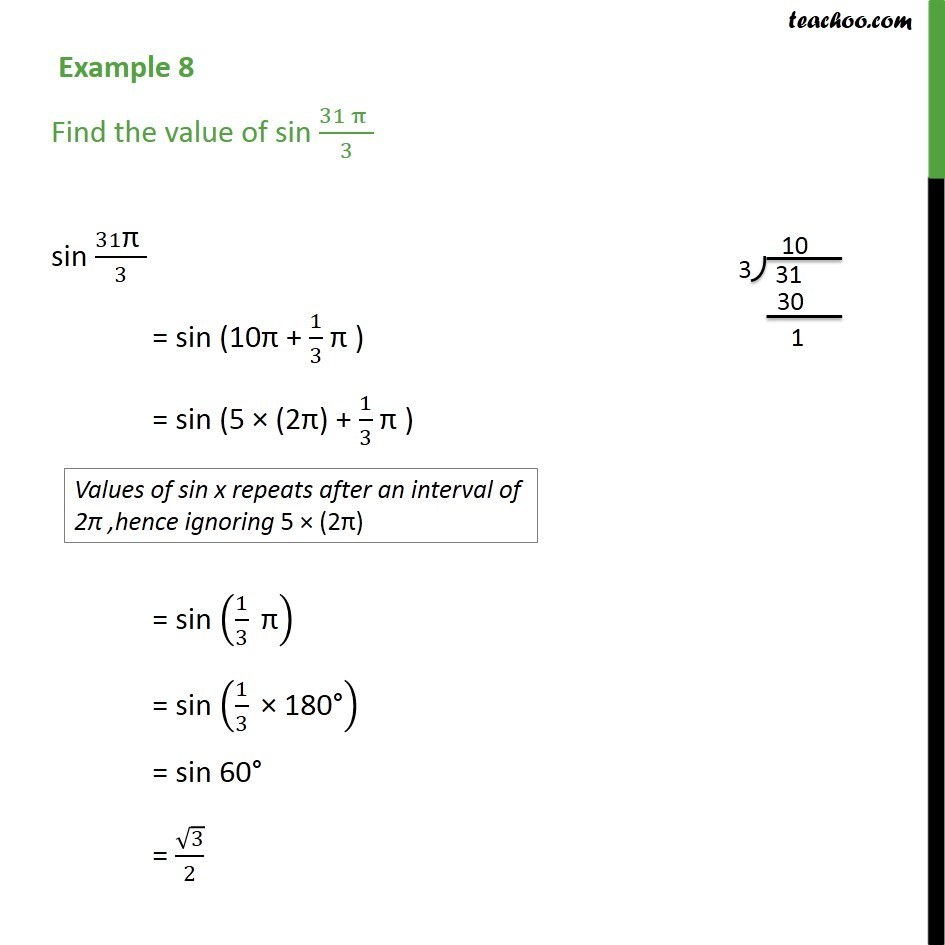1. Chapter 3 Class 11 Trigonometric Functions (Term 2)
2. Serial order wise
3. Examples

Transcript

Example 8 Find the value of sin (31" " π" " )/3 sin 31"π " /3 = sin (10π + 1/3 "π ") = sin (5 × (2π) + 1/3 "π ") = sin (1/3 " π" ) = sin (1/3 " × 180°" ) = sin 60° = √3/2